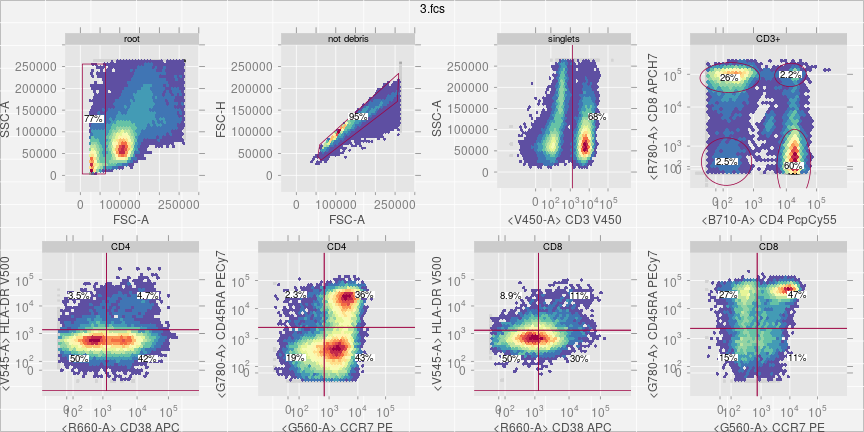# How to plot gated data

## Usage

``````plotGate(x, y, ...)
``````

## Arguments

x
GatingSet or GatingHierarchy object

y
character the node name (in the form of full or partial gating path) or numeric representing the node index in the GatingHierarchy. or missing which will plot all gates.

other arguments

## plot gating tree

``````# plot the entire gating tree
plot(gs)
# plot a sub-tree
plot(gs, "CD3+")
plot(gs, "CD4")
``````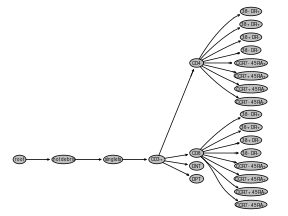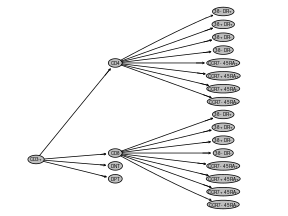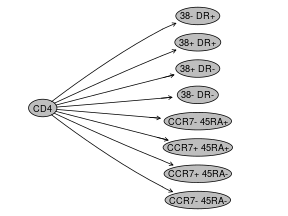## plotGate

### Change resolution

xbin The argument passed to hexbin ,which is the number of bins partitioning the range of xbnds. It is set as 0 by default,which plots all the events without binning.

sample.ratio the ratio of sub-sampling of events to speed up plotting.

``````# default xbin = 32
plotGate(gs[], "CD4/38+ DR+", main = "default (xbin = 32)")
# increase the resolution
plotGate(gs[], "CD4/38+ DR+", xbin = 128, main = "xbin = 128")
# have the highest resolution by disabling hexbin
plotGate(gs[], "CD4/38+ DR+", xbin = 0, main = "xbin = 0 (no binning)")
# speed up plotting by sub-sampling the data points
# most of them it is subsampled data is sufficient to represent the
# population distribution
plotGate(gs[], "CD4/38+ DR+", sample.ratio = 0.4, main = "sample.ratio = 0.4")
``````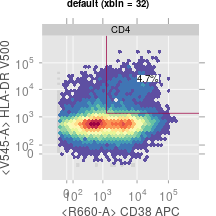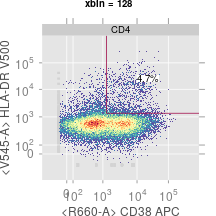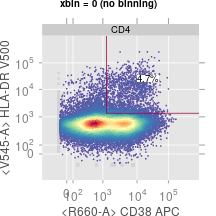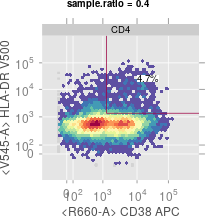### Change strip text

strip specifies whether to show pop name in strip box,only valid when x is GatingHierarchy

path A character or numeric scalar passed to gs_get_pop_paths method (used to control how the gating/node path is displayed)

``````# hide the strip
plotGate(gs[], "CD4/38+ DR+", strip = FALSE, main = "strip = FALSE")
# change the gating path length in strip
plotGate(gs[], "CD4/38+ DR+", path = "full", main = "path = 'full'")
plotGate(gs[], "CD4/38+ DR+", path = 2, main = "path = 2")
``````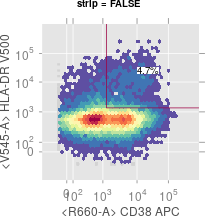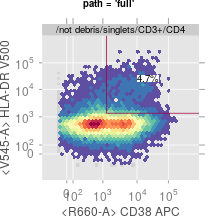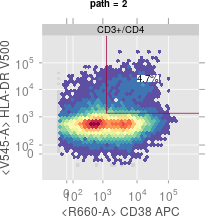### Change statistics

stats is a logical scalar indicating whether to display statistics. Default is FALSE.

pos is the numeric scalar (range within c(0,1)) or vector(length of 2,first is for x-axis,second for y-axis) to control the position of the statistics label. It is set as 0.5,which is the center.

abs is a logical scalar indicating whether the pos is relative to the gate boundary or the entire xy-axis(absolute position). By default it is set as FALSE,which indicates the position is relative to gate.

digits is an integer indicating the number of significant digits to be used when displaying the percentage of population statistics,Default is 2. see more details from format

``````# hide the pop stats
plotGate(gs[], "CD4/38+ DR+", stats = FALSE, main = "stats = FALSE")
# adjust the location, size, background of the stats
plotGate(gs[], "CD4/38+ DR+"
, pos = c(0.6, 0.9)
, digits = 4
, par.settings = list(gate.text = list(background = list(fill = "yellow") #stats background
, cex = 2 #stats font size

)
)
)
``````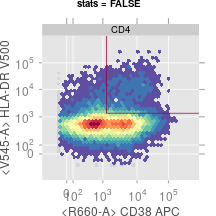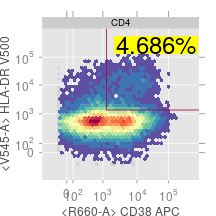### Change the scale and label text of x,y axis

marker.only specifies whether to show both channel and marker names

raw.scale whether to show the axis in raw(untransformed) scale

xlim, ylim can be either “instrument” or “data” which determines the x, y axis scale either by instrument measurement range or the actual data range. or numeric which specifies customized range.

``````# show the tranformed scale instead of raw scale
plotGate(gs[], "CD4/38+ DR+", raw.scale = FALSE, main = "raw.scale = FALSE")
# use the actual data range instead of instrument range
plotGate(gs[], "CD4/38+ DR+", xlim = "data", ylim = "data", main = "xlim = 'data'")
# zooming by manually set xlim and ylim
plotGate(gs[], "CD4/38+ DR+", xlim = c(1000, 4000), ylim = c(1000, 4000), main = "xlim = c(1000, 4000)")
# hide the channel names and only show the marker names in x,y labs
plotGate(gs[], "CD4/38+ DR+", marker.only = TRUE, main = "marker.only = TRUE")
``````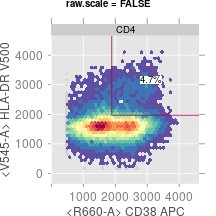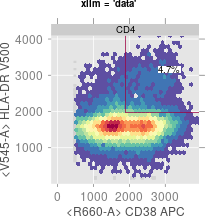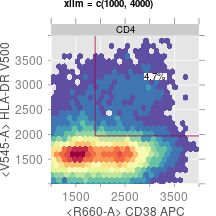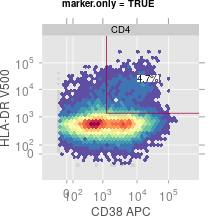### Change the lattice graphic settings of plots

par.settings list of graphical parameters passed to lattice;

``````# change the gate color and width
plotGate(gs[], "CD4/38+ DR+"
, par.settings = list(gate = list(col = "black"
, lwd = 3
, lty = "dotted")
)
)
# change the panel background and axis label size
plotGate(gs[], "CD4/38+ DR+"
, par.settings = list(panel.background = list(col = "white")
, par.xlab.text = list(cex = 1.5)
, par.ylab.text = list(cex = 1.5)
, axis.text = list(cex = 1.5)
)
)
``````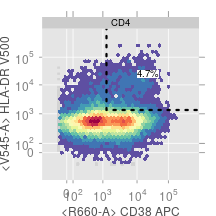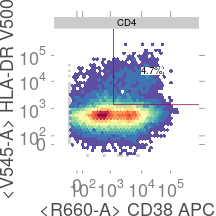### Overlay the popluations

overlay indicating the index of a gate/populationwithin the GatingHierarchy or a logical vector that indicates the cell event indices representing a sub-cell population. This cell population is going to be plotted on top of the existing gates(defined by y argument) as an overlay.

``````# display one population as an overlay on top of another population
plotGate(gs[], "CD4/CCR7- 45RA+", overlay = "CD4/38+ DR+")
plotGate(gs[], "CD4/CCR7- 45RA+"
, overlay = "CD4/38+ DR+"
, par.settings = list(overlay.symbol = list(cex = 0.1
, fill = "black"
, bg.alpha = 0.1 #dim the background density
)
)
)
plotGate(gs[], "CD4/CCR7- 45RA+"
, overlay = c("CD4/CCR7+ 45RA+", "CD4/CCR7+ 45RA-")
, par.settings = list(overlay.symbol = list(cex = 0.05, bg.alpha = 0.1))
)
# customize the symbol and legend
plotGate(gs[], "CD4/CCR7- 45RA+"
, overlay = c("CD4/CCR7+ 45RA+", "CD4/CCR7+ 45RA-")
, par.settings = list(overlay.symbol = list(cex = 0.05, bg.alpha = 0.1))
, overlay.symbol = list(`CCR7+ 45RA+` = list(fill = "black") #this overwrite the par.settings
, `CD4/CCR7+ 45RA-` = list(fill = "darkgreen")
)
, key = list(text = list(c("CCR7+ 45RA+", "CCR7+ 45RA-")) # add legend
, points = list(col = c("black", "darkgreen"), pch = 19, cex = 0.5)
, columns = 2
)
)
``````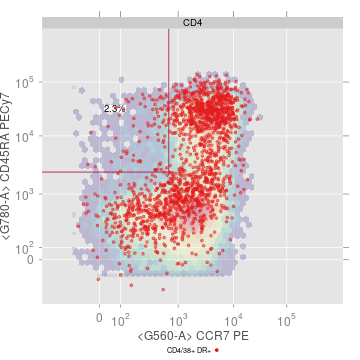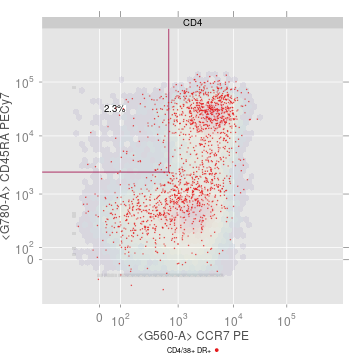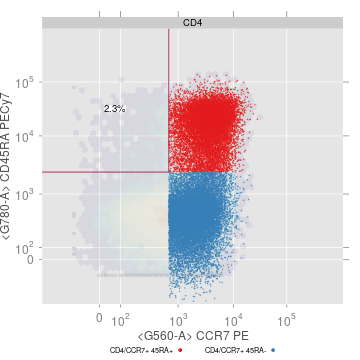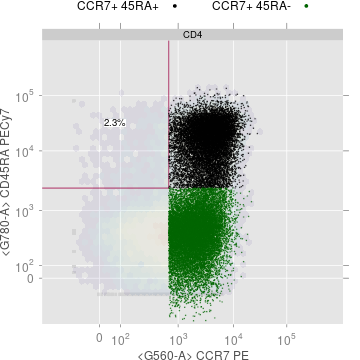### plot multiple populations

merge logical indicating whether to draw multiple gates on the same plot if these gates share the same parent population and same x,y dimensions/parameters;

arrange.main The title of the main page of the plot. Default is the sample name. Only valid when x is GatingHierarchy

gpar list of grid parameters passed to grid.layout;

arrange logical indicating whether to arrange different populations/nodes on the same page via grid.arrange call.

projections list of character vectors used to customize x,y axis. By default, the x,y axis are determined by the respective gate parameters. The elements of the list are named by the population name or path (see y). Each element is a pair of named character specifying the channel name(or marker name) for x, y axis. Short form of channel or marker names (e.g. “APC” or “CD3”) can be used as long as they can be uniquely matched to the dimentions of flow data. For example, `projections = list("lymph" = c(x = "SSC-A", y = "FSC-A"), "CD3" = c(x = "CD3", y = "SSC-A"))`

``````# plot multiple populations (gates are merged into same panel if they share the same parent and projections)
plotGate(gs[], c("CD4", "CD8"))
# flip the projection
plotGate(gs[], c("CD4", "CD8"), projections = list("CD4" = c(x = "CD8", y = "CD4")))
# dot not merge the gates
plotGate(gs[], c("CD4", "CD8"), merge = FALSE)
# use gpar to change layout
# use arrange.main to change the default title
plotGate(gs[], c("CD4", "CD8"), merge = FALSE, gpar = list(nrow = 1), arrange.main = "CD4 vs CD8")
``````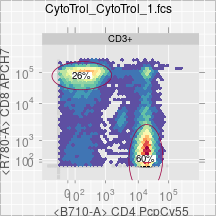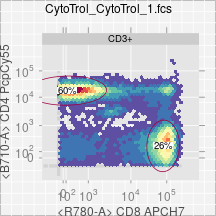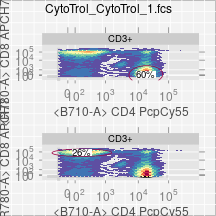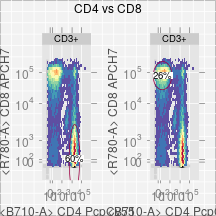``````# or return populations as individual trellis objects
#and arrange them manually
latticeObjs <- plotGate(gs[], c("CD4", "CD8"), merge = FALSE, arrange = FALSE)
do.call(grid.arrange, c(latticeObjs, nrow = 1, main = "Tcell subsets"))
``````
``````## Warning: 'clone' is deprecated.
## See help("Deprecated")

## Warning: 'clone' is deprecated.
## See help("Deprecated")

## Warning: 'clone' is deprecated.
## See help("Deprecated")

## Warning: 'clone' is deprecated.
## See help("Deprecated")
``````

### Facetting on study variables

cond the conditioning variable to be passed to lattice plot.

``````# condition on `Stim` and `PTID`
plotGate(gs, "CD4/38+ DR+", cond = "Stim+PTID")
# to put the second condition variable to the y axis
latticeExtra::useOuterStrips(plotGate(gs, "CD4/38+ DR+", cond = "Stim+PTID"))
``````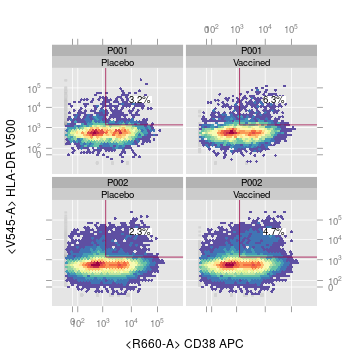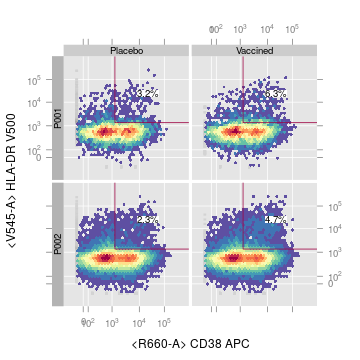### Plot 1-d gate

type either `xyplot` or `densityplot`. Default is `xyplot` default.y specifiying y channel for xyplot when plotting a 1d gate. Default is `SSC-A`. fitGate used to disable behavior of plotting the gate region in 1d densityplot stack whether to stack all samples (vetically) in one panel

``````plotGate(gs[c(1,3)], "CD3+")
# change the default y axis
plotGate(gs[c(1,3)], "CD3+", default.y = "<B710-A>")
# change the plot type
plotGate(gs[c(1,3)], "CD3+", type = "densityplot")
# fit the 1d gate onto 1d density
plotGate(gs[c(1,3)], "CD3+", type = "densityplot", fitGate = TRUE)
# stack them together
plotGate(gs[c(1,3)], "CD3+", type = "densityplot", stack = TRUE)
``````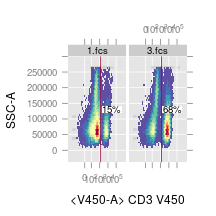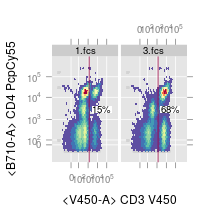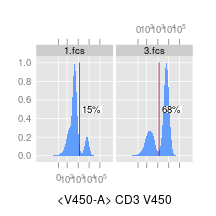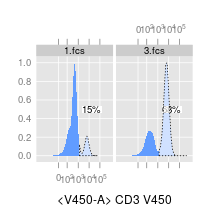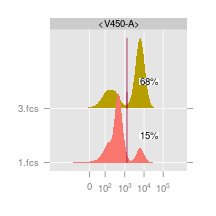### Plot all the gates

``````plotGate(gs[], gpar = list(nrow = 2))
``````